Next: 4.2 Mirror plus PSPC Up: 4.1 Spatial Resolution Previous: Focus and Penetration Effects

## Gaussian Intrinsic Resolution and Mirror Blur Term

Due to the inherent statistics of the primary electron generation, the intrinsic spatial resolution of the ROSAT PSPC is expected to be a Gaussian.   Theoretically its width is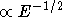and independent of. All photons not in the other two components are assumed to be in this component. Thus the fraction of photons within this component as a function of energy is given by: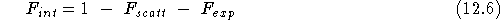Note that this becomes zero above 2 keV from equation 10.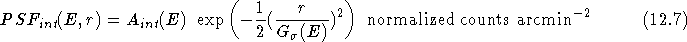The derived analytical form for the width of the detector gaussian,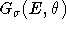, as a function of energy is given by: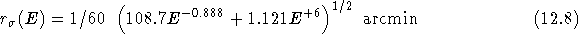The analytical form for the Gaussian mirror blur is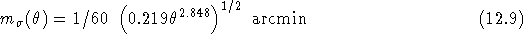These two Gaussian widths are combined as follows: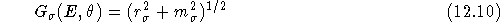The normalization,, is given by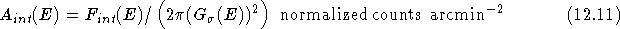where the denominator is the renormalization factor such that the integral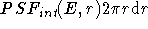over the range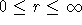equals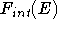.

This model has been tested against in-flight data and the model and test results are written up in [Hasinger et al.1993].

If you have problems/suggestions please send mail to rosat_svc@mpe-garching.mpg.de